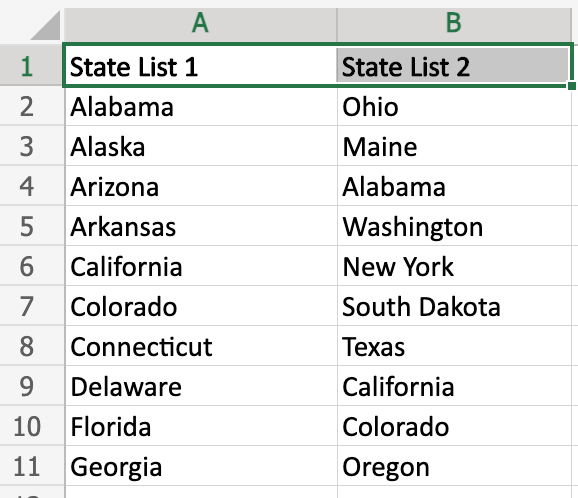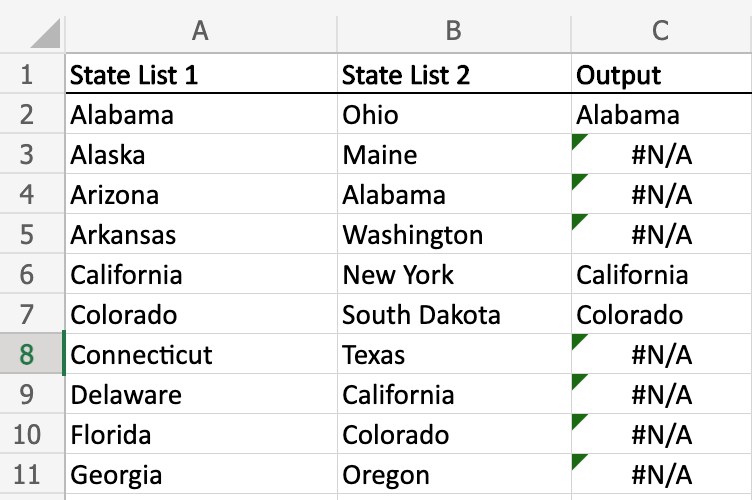#### How to VLOOKUP Another Column?

Working with large sets of data can often be intimidating. There may be times when you need to identify one column of data compared to another, either to quickly identify which values are missing, or to pull out specific cells. So how do you do that without manually reviewing each cell? The answer – VLOOKUP.

You need to know how to use vlookup in Excel. If you’re unfamiliar with VLOOKUP, this short guide will give you some quick tips on how to VLOOKUP another column in an Excel Worksheet.

## Why Use VLOOKUP to Compare Columns?

There are a few common reasons to use VLOOKUP to compare columns – we will focus on using VLOOKUP to identify missing or different values between two columns. As we can see in our example, we have two lists of states. We can use VLOOKUP to quickly identify which states are not on both lists. You can follow this using learning materials.## The Vlookup formula

Rather than manually reviewing each list, we can use the VLOOKUP formula to flag states that do not appear on both lists. In cell C4, let’s start entering the VLOOKUP formula. To identify states that do not appear on both lists, we will use the following formula:

=VLOOKUP(A2,B:B,1,FALSE)

This formula is pointing to states in List 1, checking if they are in Column B, and identifying any states that are in both lists in Column C.

To break this down further, A2 is pointing to Alabama in State List 1. The formula is identifying Alabama to search in our range of data we identify, which is Column B (B:B). Since we are only searching in one column, we simply put 1 as the column index number. Finally, we need to include FALSE as the range_lookup value so it only shows exact matches when comparing the lists.When you enter the VLOOKUP formula, any state names that appear in the output column (Column C) are present in both lists. Any cells that show #N/A mean that the state in the same row in Column A is not present in Column.

By using the VLOOKUP function to look up data in a different column, you can easily find and return data from a table, even if it’s not in the first column. With just a few changes to the column number argument, you can look up data from any column in the table.

## Using VLOOKUP to Compare Columns with Different Data Types

In the previous chapter, we saw how to use VLOOKUP to compare columns that contain the same data type. However, what if the columns contain different data types? For example, what if one column contains text and the other column contains numbers?

In this case, we can use the MATCH function in conjunction with VLOOKUP. The MATCH function has three arguments:

• lookup_value: The value that you want to find in the range.
• range_array: The range of cells that contains the data that you want to search.
• match_type: A number that specifies the type of match that you want to find.
• The MATCH function will return the position of the lookup_value in the range_array. The position is a number, starting from 1.

We can use the MATCH function to find the column index number of the column that contains the value that we want to return. For example, if we want to return the state name from column B that matches the state name in cell A2, we can use the following formula:

`=MATCH(A2,B:B,0)`

The MATCH function will return the position of the state name in cell A2 in column B. The 0 in the match_type argument specifies that we want an exact match.

Now that we know the column index number of the column that contains the value that we want to return, we can use VLOOKUP to return the value. The following formula will return the state name from column B that matches the state name in cell A2:

`=VLOOKUP(A2,B:B,MATCH(A2,B:B,0),FALSE)`

This formula is the same as the formula in the previous chapter, except that we have added the MATCH function to the first argument.

The MATCH function allows us to use VLOOKUP to compare columns that contain different data types. This can be useful in a variety of situations, such as when you need to compare customer names and order numbers, or when you need to compare product names and prices.